JEE  >  Test: Laws of Motion - 2

# Test: Laws of Motion - 2

Test Description

## 25 Questions MCQ Test Physics Class 11 | Test: Laws of Motion - 2

Test: Laws of Motion - 2 for JEE 2023 is part of Physics Class 11 preparation. The Test: Laws of Motion - 2 questions and answers have been prepared according to the JEE exam syllabus.The Test: Laws of Motion - 2 MCQs are made for JEE 2023 Exam. Find important definitions, questions, notes, meanings, examples, exercises, MCQs and online tests for Test: Laws of Motion - 2 below.
Solutions of Test: Laws of Motion - 2 questions in English are available as part of our Physics Class 11 for JEE & Test: Laws of Motion - 2 solutions in Hindi for Physics Class 11 course. Download more important topics, notes, lectures and mock test series for JEE Exam by signing up for free. Attempt Test: Laws of Motion - 2 | 25 questions in 50 minutes | Mock test for JEE preparation | Free important questions MCQ to study Physics Class 11 for JEE Exam | Download free PDF with solutions
 1 Crore+ students have signed up on EduRev. Have you?
Test: Laws of Motion - 2 - Question 1

### A student calculates the acceleration of m1 in figure shown as a1 =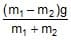. Which assumption is not required to do this calculation.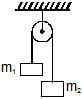Detailed Solution for Test: Laws of Motion - 2 - Question 1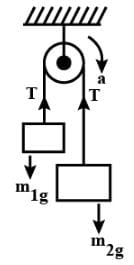Here, m1​a=T−m1​g...(1)
m2​a=m2​g−T...(2)
(1)+(2),a=m1​+m2​m2​−m1​​g
Acceleration of m1​, a1​=−a= ​m1​−m2​​g/ m1​+m2
For this calculation, we assume that the string is massless and rim of the pulley is frictionless otherwise there would have been a frictional force and mass of string included in the acceleration expression. The tension will come due to the inextensible string, otherwise. the elastic effect would have to be included in the tension. The mass of the pulley does not affect the given expression as tension on both the sides remain the same. Thus, option C is correct.

Test: Laws of Motion - 2 - Question 2

### Which graph shows best the velocity-time graph for an object launched vertically into the air when air resistance is given by |D| = bv? The dashed line shows the velocity graph if there were no air resistance.

Detailed Solution for Test: Laws of Motion - 2 - Question 2

Let the mass of object launched is m, the acceleration due to gravity is g and the acceleration of the object at any instant = a
Using Newton’s second law of motion,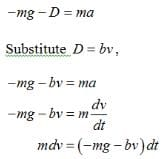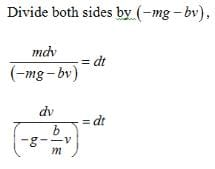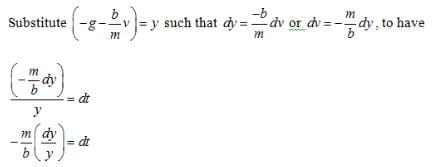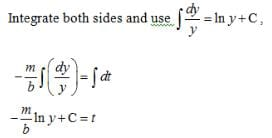So,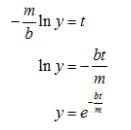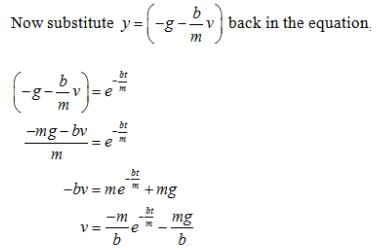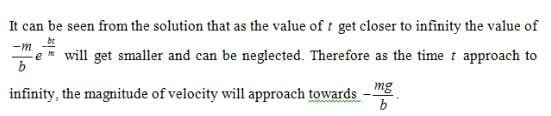Only option B follows the above discussion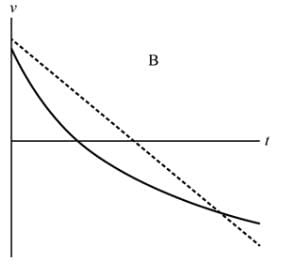*Multiple options can be correct
Test: Laws of Motion - 2 - Question 3

### Two men of unequal masses hold on to the two sections of a light rope passing over a smooth light pulley. Which of the following are possible?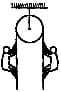Detailed Solution for Test: Laws of Motion - 2 - Question 3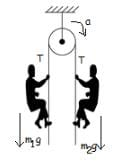Here m2​>m1
If lighter man is stationary,
Vertical force on m1​
T−m1​g=0
⇒T=m1​g
Vertical force on m2
m2a=T−m2​g=m1​g−m2​g
a= ​m1/ m2 ​​g−g
since  ​m1/ m2​​<1, so in this case, a is negative. thus the heavier man of mass m2​ is sliding down.
If heavier man is stationary,
Vertical force on m2​=T−m2​g=0
⇒T=m2​g
Vertical force on m1​=m1​a=T−m1​g=m2​g−m1​g
a= ​m2/ m1 ​​g−g
since m2​>m1​ so in this case, a is positive . Thus the lighter man will climb up with some acceleration.
From the above two cases we can say that they will move with the same acceleration over the pulley but in the opposite direction.
Ans:(A),(B),(D)

Test: Laws of Motion - 2 - Question 4

Adjoining figure shows a force of 40 N acting at 30° to the horizontal on a body of mass 5 kg resting on a smooth horizontal surface. Assuming that the acceleration of free-fall is 10 ms_2, which of the following statements A, B, C, D, E is (are) correct?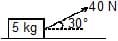The horizontal force acting on the body is 20 N

 The weight of the 5 kg mass acts vertically downwards

 The net vertical force acting on the body is 30 N

Detailed Solution for Test: Laws of Motion - 2 - Question 4

(C) 2 only

 The weight of the 5kg mass acts vertically downwards

*Multiple options can be correct
Test: Laws of Motion - 2 - Question 5

For ordinary terrestrial experiments, which of the following observers below are inertial.

Detailed Solution for Test: Laws of Motion - 2 - Question 5

For an inertial frame the acceleration of the object should be = 0

*Multiple options can be correct
Test: Laws of Motion - 2 - Question 6

In the system shown in the figure m1 > m2. System is held at rest by thread BC. Just after the thread BC is burnt :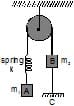Detailed Solution for Test: Laws of Motion - 2 - Question 6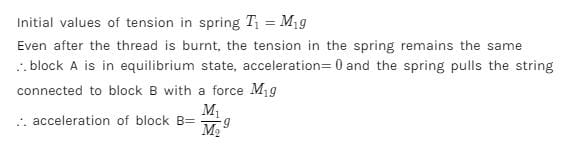*Multiple options can be correct
Test: Laws of Motion - 2 - Question 7

A particle is resting on a smooth horizontal floor. At t = 0, a horizontal force starts acting on it. Magnitude of the force increases with time according to law F = a.t, where a is a constant. For the figure shown which of the following statements is/are  correct ?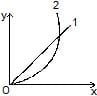Detailed Solution for Test: Laws of Motion - 2 - Question 7

a=F/m=αt/m……(i)
or a ∝ t
i.e. a-t graph is a straight line passing through
origin.
If u=0, then integration of Eq. (i) gives,
v=αt2/2m or v∝t2
Hence in this situation (when u=0) v-t graph is a
parabola passing through origin.

Test: Laws of Motion - 2 - Question 8

A cyclist of mass 30 kg exerts a force of 250 N to move his cycle. acceleration is 4 ms−2. force of friction between road and tyres will be

Detailed Solution for Test: Laws of Motion - 2 - Question 8

Force - force of friction = mass × acceleration
250 - f = 30 × 4
f = 250 – 120
= 130N

*Multiple options can be correct
Test: Laws of Motion - 2 - Question 9

Figure shows the displacement of a particle going along the x-axis as a funtion of time :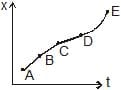Detailed Solution for Test: Laws of Motion - 2 - Question 9

For force to be zero net acceleration should be zero i.e. velocity should remain constant. In an x-t graph if slope is a straight line it means that velocity is constant which is the case for regions AB, BC and CD.

*Multiple options can be correct
Test: Laws of Motion - 2 - Question 10

A force of magnitude F1 acts on a particle so as to accelerate it from rest to a velocity v. The force F1 is then replaced by another force of magnitude F2 which decelerates it to rest.

Detailed Solution for Test: Laws of Motion - 2 - Question 10

It not a necessity that F2 must be or must not be equal to F1. If F2 is opposite in direction but smaller/larger in magnitude, it will still stop the particle.

*Multiple options can be correct
Test: Laws of Motion - 2 - Question 11

A board is balanced on a rough horizontal semicircular log. Equilibrium is obtained with the help of addition of a weight to one of the ends of the board when the board makes an angle q with the horizontal. Coefficient of friction between the log and the board is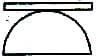Detailed Solution for Test: Laws of Motion - 2 - Question 11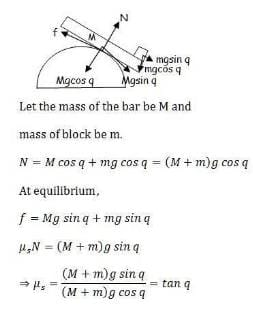Test: Laws of Motion - 2 - Question 12

A stationary body of mass m is slowly lowered onto a massive platform of mass M (M >> m) moving at a speed V0 = 4 m/s as shown in fig. How far will the body slide along the platform (μ = 0.2 and g = 10 m/s2)?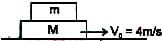Detailed Solution for Test: Laws of Motion - 2 - Question 12

If we move relative to the block, then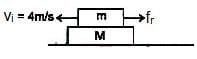Now, fr = μmg
a = μg
a = - 2 m/s2 (i.e. towards right)
The final velocity of the small block is 0 so
2as = vf2 – vi2
-4s = -16
s = 4m

*Multiple options can be correct
Test: Laws of Motion - 2 - Question 13

A block of mass 2.5 kg is kept on a rough horizontal surface. It is found that the block does not slide if a horizontal force less than 15 N is applied to it. Also it is if found that it takes 5 second to slide throughout the first 10 m if a horizontal force of 15 N is applied and the block is gently pushed to start the motion. Taking g = 10 m/s2, then

Detailed Solution for Test: Laws of Motion - 2 - Question 13

The weight of the mass of block, m = 2.5 kg
Now comparing the values of the weight of block = size of Normal force = mg = (2.5)(10) = 25 N
The horizontal force applied = 15 N (capable to move blocks)
{where d = 10 m, and t = 5 s, and "a" = block's acceleration}
The net force acting on the block of weight = ma = (2.5)(0.8) = 2.0 N
The force required to oppose the external force = 15 - 2 = 13 N
The value of the Kinetic Friction Force = 13 N
Therefore, dividing the Kinetic Force Friction and Horizontal Force by Normal Force we get
Coefficient of kinetic friction = 13/25 = 0.52 ANS
Coefficient of static friction = 15/25 = 0.60 ANS

*Multiple options can be correct
Test: Laws of Motion - 2 - Question 14

The coefficient of friction between 4 kg and 5 kg blocks is 0.2 and between 5 kg block and ground is 0.1 respectively. Choose the correct statements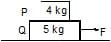Detailed Solution for Test: Laws of Motion - 2 - Question 14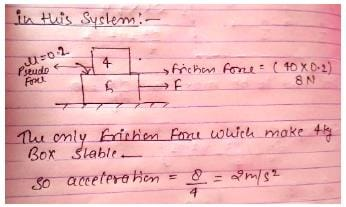Test: Laws of Motion - 2 - Question 15

In a tug-of-war contest, two men pull on a horizontal rope from opposite sides. The winner will be the man who

Detailed Solution for Test: Laws of Motion - 2 - Question 15

The greater the force exerted on the ground, the greater is the reaction and therefore the greater pull will be given to the rope.

*Multiple options can be correct
Test: Laws of Motion - 2 - Question 16

A man pulls a block heavier than himself with a light horizontal rope. The coefficient of friction is the same between the man and the ground, and between the block and the ground.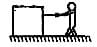Detailed Solution for Test: Laws of Motion - 2 - Question 16

The friction force between the block and ground is more as compared to friction force between man and ground.
such that unless man doesn't move the block will not be moved.
The block of mass say M is heavier than the man of mass say m. The surface is rough with friction coefficient say μ. So when the man applies the force on the block the force cannot exceed the frictional force μmg without moving as μMg>μmg. Now if he starts moving (i.e. the force applied is increased and now the friction between him and the surface is not holding him stationary) there is a possibility that the block may move. Now as there is no other force acting on the system and as the man is lighter than block so he would have greater acceleration than the block when both move.

*Multiple options can be correct
Test: Laws of Motion - 2 - Question 17

In figure, two blocks M and m are tied together with an inextensible and light string. The mass M is placed on a rough horizontal surface with coefficient of friction m and the mass m is hanging vertically against a smooth vertical wall. The pulley is frictionless.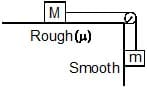Choose the correct statement(s)

Detailed Solution for Test: Laws of Motion - 2 - Question 17

The friction force on the mass M due to rough surface is f = μMg
In equilibrium,
For mass m,
T = mg,
for mass M,
f = μMg = T
⇒ mg = μMg
⇒ m = μM
If mg > μMg, then there would be an acceleration.
Thus, we get m > μM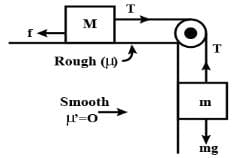Test: Laws of Motion - 2 - Question 18

When a net force act on a body, it produces acceleration in body in direction of net force which is directly proportional to net force acting on body and inversely proportional to its mass. This statement is called

Detailed Solution for Test: Laws of Motion - 2 - Question 18

That is the statement of Newton's 2nd law of motion.

*Multiple options can be correct
Test: Laws of Motion - 2 - Question 19

Imagine a situation in which the horizontal surface of block M0 is smooth and its vertical surface is rough with a coefficient of friction m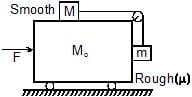Identify the correct statement(s)

Detailed Solution for Test: Laws of Motion - 2 - Question 19

If no force is applied, the block A will slip on C towards right and the block B will move downward. Suppose the minimum force needed to prevent slipping is F, taking A+B+C as the system, the only external horizontal force on the system is F. Hence the acceleration of the system is
a = F/2m+M
take block A as the system. The force on A are
1) Tension T by the string towards right
2) Friction f by the block C towards left
3) Weight mg downward and
4) Normal force N upward.
For vertical equilibrium N = mg.
As the minimum force needed to prevent slipping is applied, the friction is limiting thus
f = μN = μmg
As the block moves towards right with an acceleration a,
T –f = ma
Or T - μmg = ma                                                               ----------------- (2)
Now take the block B as the system the forces are as shown in fig (b).
1) Tension T upward.
2) Weight mg downward
3) Normal force N1 towards right
4) Friction f1 upward.
As the block moves towards right with an acceleration a, N = ma.
As the friction is limiting, f1= μN’ = μma
For vertical equilibrium
T + f1 = mg
T + μma = mg
Eliminating T from (1) and (3)
amin = (1- μ)*g/(1+ μ)
When a large force is applied the block A slips on C-towards left and the block B slips on C in the upward direction. The friction on A is towards right and that on B is downwards solving as above, the acceleration in this case is
Thus, the force should be between
(1- μ)(M+2m)*g/(1+ μ) and
(1+ μ)(M+2m)*g/(1- μ)

*Multiple options can be correct
Test: Laws of Motion - 2 - Question 20

In above problem, choose the correct value(s) of F which the blocks M and m remain stationary with respect to M0

Test: Laws of Motion - 2 - Question 21

In the figure the tension in the diagonal string is 60 N.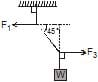. Find the magnitude of the horizontal force and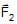that must be applied to hold the system in the position shown.

Detailed Solution for Test: Laws of Motion - 2 - Question 21

The diagram should be changed to the following: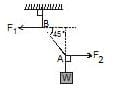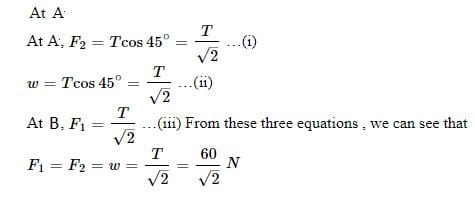*Multiple options can be correct
Test: Laws of Motion - 2 - Question 22

In the above questions what is the weight of the suspended block ?

Detailed Solution for Test: Laws of Motion - 2 - Question 22

The question is incomplete and is too vague to be found
It should be removed so as to not cause confusion.

*Multiple options can be correct
Test: Laws of Motion - 2 - Question 23

A particle of mass m is constrained to move on x-axis. A force F acts on the particle. F always points toward the position labeled E. For example, when the particle is to the left of E, F points to the right. The magnitude of F is constant except at point E where it is zero.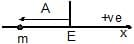The system is horizontal. F is the net force acting on the particle. The particle is displaced a distance A towards left from the equilibrium position E and released from rest at t = 0

What is the period of the motion ?

Detailed Solution for Test: Laws of Motion - 2 - Question 23

Since the force is uniform the particle takes equal time to got to E from P and to Q from E. Now if T is the time taken by it to go to E from P then the particle takes 4 such time periods to come back to its original position.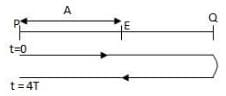F=ma ⇒ a=F/m
If time taken by the particle to move from p to E is t then,
A=1/2 aT2=1/2(F/m)T2
⇒T= √2am/F
Time taken by the particle to come back to P is t=4T
⇒ t=4(√2Am/F)

*Multiple options can be correct
Test: Laws of Motion - 2 - Question 24

Velocity-time graph of the particle is

Detailed Solution for Test: Laws of Motion - 2 - Question 24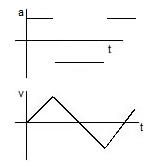In the velocity time graph, the slope value should give the value of acceleration.

*Multiple options can be correct
Test: Laws of Motion - 2 - Question 25

Find minimum time it will take to reach from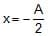to 0.

Detailed Solution for Test: Laws of Motion - 2 - Question 25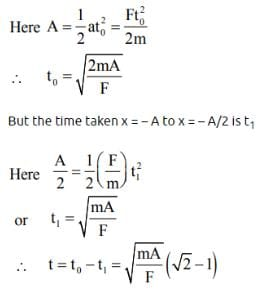## Physics Class 11

127 videos|464 docs|210 tests
 Use Code STAYHOME200 and get INR 200 additional OFF Use Coupon Code
Information about Test: Laws of Motion - 2 Page
In this test you can find the Exam questions for Test: Laws of Motion - 2 solved & explained in the simplest way possible. Besides giving Questions and answers for Test: Laws of Motion - 2, EduRev gives you an ample number of Online tests for practice

## Physics Class 11

127 videos|464 docs|210 tests